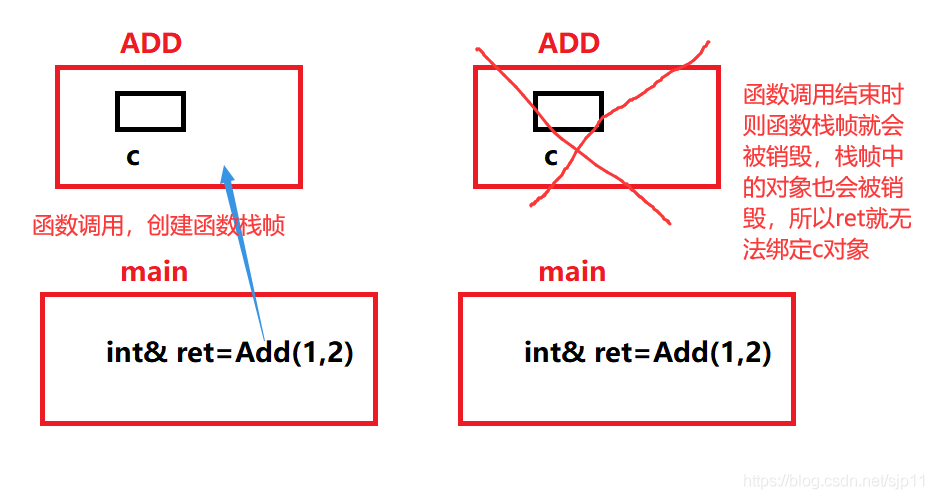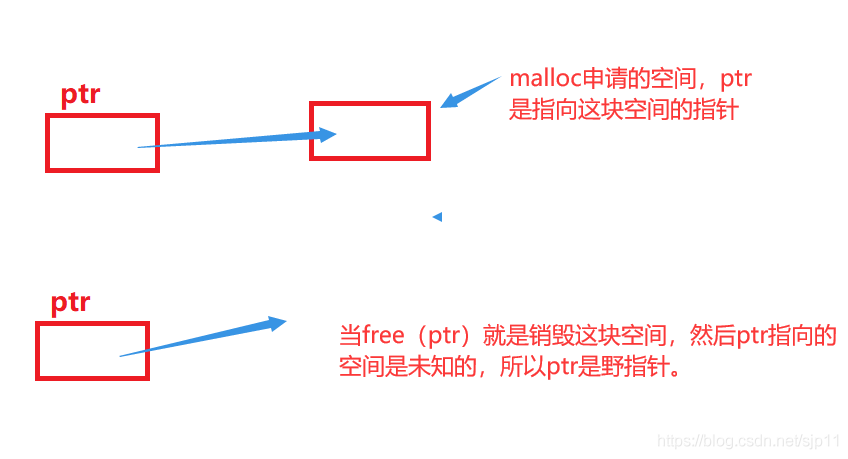﻿ c++基础学习之如何区分引用和指针_C 语言_脚本之家
C 语言c++基础学习之如何区分引用和指针

C语言中只有指针,C++加入了引用,能够起到跟指针类似的作用,下面这篇文章主要给大家介绍了关于c++基础学习之区分引用和指针的相关资料,文中通过示例代码介绍的非常详细,需要的朋友可以参考下

前言

c++程序员们在很多场合都会使用对象这个名词，通常情况下，对象是指一块能存储数据并具有某种类型的内存空间。有些人仅在与类有关的场景下才使用“对象”这个词。在这里，即认为对象是具有某种数据类型的内存的空间

1.引用

1.1引用的概念

int i = 10;
int& refi = i;//refi是i的另外一个名字
int& refi2;//报错：引用必须初始化//refi,refi1,refi2与i绑定在一起
int& refi1 = i;
int& refi2 = refi1;

refi = 100;//将100的值赋值给refi，即把值给i
int j=refi;//与int j=i;是一样的

1.2引用的定义

int i = 10, int i1 = 10;//i和i1都是int型
int& r = i, int i1 = i;//r是引用，与i进行绑定，i1是int
int i3 = i1, & r2 = i1;//i3是int，r2是引用，与i1绑定一起
int& r3 = i, & r4 = i;//r3和r4都是引用

int& refi = 10;//错误：引用类型的初始值必须是一个对象
int i = 10;
double& d = i;//错误：引用的类型必须是int型

1.3引用与const

const int i = 10;
const int& r1 = i;//正确：引用及其对应的对象都是常量
const int& r2 = 200;//正确：引用及其对应的对象都是常量
r1 = 40;//错误：r1是常量的引用，不能修改
int& r2 = r1;//错误：r1是常量引用，只能读，r2是非常量引用，能读能修改

int i = 42;
const int& r1 = i;//正确：允许将const int&绑定到一个普通int对象上
const int& r2 = i * 2;//正确：r2是一个常量引用
int& r3 = i * 2;//错误:r3是一个普通的非常量引用

double d1 = 1.111;
const int& r3 = d1;

const int tmp = d1;//由double生成一个int类型的临时量
const int& r3 = tmp;//让r3绑定这个临时量tmp

int i = 10;
int& r1 = i;
const int& r2 = i;//正确：const引用可以引用非const对象，但是不能通过r2修改i
r1 = 20;//正确：r1为非const引用，可以修改
r2 = 30;//错误：r2为cosnt引用，不能对i进行修改

1.4引用的使用场景

1.做参数

void Swap(int& left, int& right)
{
int temp = left;
left = right;
right = temp;
}

int main（）
{
int a=0，b=10；
swap（a,b);
}

2.做返回值

int& Count()
{
static int n = 0；//n存在静态区中
n++;
return n; //返回对象是n的引用
}

{
int c = a + b;

return c;
}

int main()
{
//这是错误的：因为c出了函数作用域后则会被销毁，则c这块空间就不存在
//ret引用就无效。
}2.指针

2.1概念

int* i1, * i2;//i1和i2都是int类型的指针
int d, * d1;//d是int对象，d1是指向int类型的指针

2.2获取对象的地址

int i = 10;
int* pi = &i;//pi存放变量的i的地址，或者说pi是指向变量i的指针注意:指针也是有大小的，它的大小根据在不同的平台是不同的，与指针的类型无关。

double d;
double* pd = &d;//正确：pd是指向double对象的指针
int* pi1 = &d;//错误：试图将double对象的地址给int类型的指针
pi1=pd;//错误：指针pd和pi1的类型不匹配

2.3利用指针访问对象

int i = 10;
int* pi = &i;//pi存放变量的i的地址，或者说pi是指向变量i的指针

*pi = 20;//将i的值修改为20
cout << *pi << endl;//输出的是i对象输出20

2.3空指针

int* p1 = nullptr;//等价于int *p1=0;
int* p2 = 0;//直接将p2初始化为字面常量0
//需要先#inclde cstdlib
int* p3 = NULL;

int zero = 0;
int* p4 = zero;//错误：不能将int变量赋值给指针

2.4野指针

2.4.2野指针的产生：

int* p1;//指针没有初始化
int arr = { 0 };
arr = 11;//指针的越界访问
int* ptr = (int*)malloc(sizeof(int));
free(ptr);//指针指向的空间被释放

1.指针未初始化：

2.指针的越界访问：

3. 指针指向的空间释放：2.5各个指针类型的含义

int n = 10;
char* pc = (char*)&n;//pc为char类型的指针，但它指向n
int* pi = &n;//pi为int类型的指针，指向n

printf("%p\n", &n);//输出结果：012FF7E0
printf("%p\n", pc);//输出结果：012FF7E0
printf("%p\n", pc + 1);//输出结果：012FF7E1
printf("%p\n", pi);//输出结果：012FF7E0
printf("%p\n", pi + 1);//输出结果：012FF7E4

//11223344是16进制数字,0x是16进制的标识符，每两个数字是一个字节
int n = 0x11223344;
char *pc = (char *)&n;//pc为char类型的指针，指向n
int *pi = &n;//pi为int类型指针，指向n
*pc = 0;   //i变为0x11223300
*pi = 0;   //i变为0x00000000

pi和pc存放的都是n的地址，pc是char类型的指针，通过pc访问n的时候只能访问1个字节，所以*pc只改变一个字节的数值，pi是int类型的指针，通过pi访问n的时候只能访问4个字节，所以*pc改变4个字节的数值.

2.6 void* 指针

void*指针是一种特殊类型的指针，它可存放任意类型的指针.但我们无法确定对该地址中到底是个什么类型的对象。所以我们不能直接操作void*指针所指的对象。

double d;
void* pv = &d;//正确，d可以是任意类型的对象

2.7指向指针的指针

通过*的个数可以区别指针的级别，例如 **表示指向指针的指针，***表示指向指针的指针指针。

int i = 0;
int* pi = &i;
int** ppi = &pi;//ppi指向pi的指针
*ppi;//*ppi等于pi
**ppi = 10;//**pi等于i2.8指针与const

const int i = 10;
int* pi = &i;//错误：pi是一个普通的指针
const int* pi1 = &i;//正确：pi1是一个指向常量的指针
*pi1 = 20;//错误：pi1指向的值不能修改

int i1 = 10;
pi1 = &i1;//正确：但不能通过pi1修改i1的值

int i2 = 0;
int i3-10；
int* const pi2 = &i2;//pi2一直指向i2
pi2=&i3;//错误：pi2是一个常量指针
*pi2=12；//正确：pi2指向的值可以被修改
const double d = 1.111;
const double* const pd = &d;//pd是一个指向常量的常量指针
*pd=2.22；//错误：pd指向的值不能被修改const在*之前是修饰指针指向的值，即指针指向的值不能被修改，const在*之后是修饰指针本身，即指针不能被修改。

3.指针和引用的区别

1.指针就是一个对象，允许对指针赋值和拷贝，引用是给对象多起一个名字，不创建对象。

2.指针定义时可以不初始化，引用定义时必须初始化。

3.引用初始化完成后，引用将和它绑定的初始值一直绑定在一起。指针可以先后指向几个不同的对象。

4.有多级指针，但没有多级引用。

5. 引用自加即引用的实体增加1，指针自加即指针向后偏移一个类型的大小